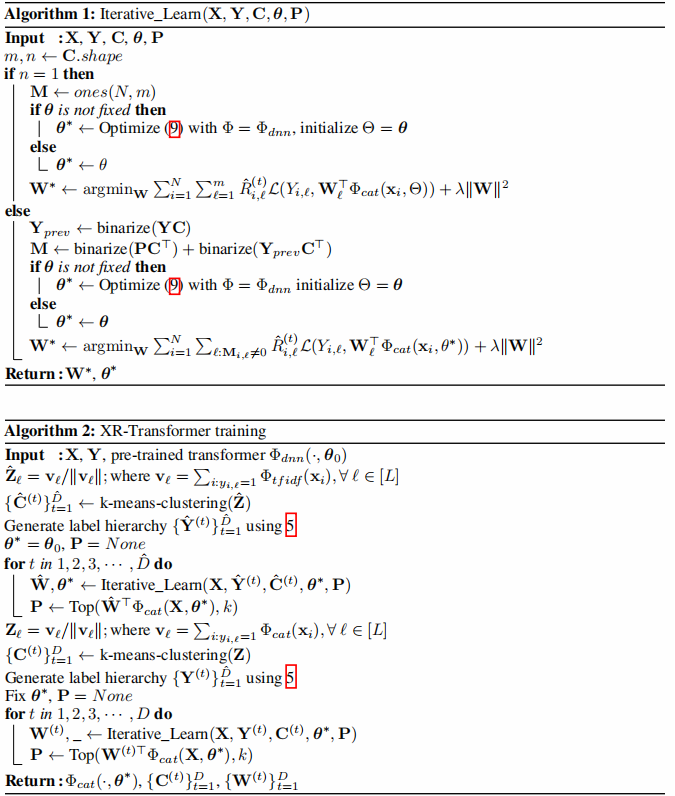# Paper Information

Title：Fast Multi-Resolution Transformer Fine-tuning for Extreme Multi-label Text Classification
Authors：Jiong Zhang, Wei-Cheng Chang, Hsiang-Fu Yu, I. Dhillon
Sources：2021, ArXiv
Other：3 Citations, 61 References

# 1 背景知识

训练集 $\left\{\mathbf{x}_{i}, \mathbf{y}_{i}\right\}_{i=1}^{N}$，$\mathbf{x}_{i} \in \mathcal{D}$ 代表着第 $i$ 个文档，$\mathbf{y}_{i} \in\{0,1\}^{L}$ 是第$i$个样本的第 $\ell$ 个标签。

eXtreme Multi-label Text Classification (XMC) 目标是寻找一个这样的函数 $f: \mathcal{D} \times[L] \mapsto \mathbb{R}$，$f(x，\ell)$ 表示输入 $x$ 与标签 $\ell$ 之间的相关性。

实际上，得到 $top-k$ 个最大值的索引作为给定输入 $x$ 的预测相关标签。最直接的模型是一对全(OVA)模型：

$f(\mathbf{x}, \ell)=\mathbf{w}_{\ell}^{\top} \Phi(\mathbf{x}) ; \ell \in[L]\quad\quad\quad(1)$

其中

• $\mathbf{W}=\left[\mathbf{w}_{1}, \ldots, \mathbf{w}_{L}\right] \in \mathbb{R}^{d \times L}$ 是权重向量
• $\Phi(\cdot)$ 是一个文本向量转换器，$\Phi: \mathcal{D} \mapsto \mathbb{R}^{d}$用于将 $\mathbf{x}$转换为 $d$ 维特征向量

为了处理非常大的输出空间，最近的方法对标签空间进行了划分，以筛选在训练和推理过程中考虑的标签。特别是 [7, 12, 13, 34, 35, 39] 遵循三个阶段的框架：partitioning、shortlisting 和 ranking。

首先 partitioning 过程，将标签分成 $K$ 个簇 $\mathbf{C} \in\{0,1\}^{L \times K}$ ，$C_{\ell, k}=1$ 代表这标签 $\ell$ 在第 $k$ 个簇中。

然后 shortlisting 过程，将输入 $x$ 映射到相关的簇当中：

$g(\mathbf{x}, k)=\hat{\mathbf{w}}_{k}^{\top} \Phi_{g}(\mathbf{x}) ; k \in[K]\quad\quad\quad(2)$

最后 ranking 过程，在 shortlisted 上训练一个输出大小为 $L$ 的分类模型：

$f(\mathbf{x}, \ell)=\mathbf{w}_{\ell}^{\top} \Phi(\mathbf{x}) ; \ell \in S_{g}(\mathbf{x})\quad\quad\quad(3)$

其中 $S_{q}(\mathbf{x}) \subset[L]$ 是标签集的一个子集。

对于基于 transformer 的方法，主要花费的时间是 $\Phi(\mathbf{x})$ 的评价。但是 $K$ 值太大或太小仍然可能会有问题。实证结果表明，当 cluster 的大小 $B$ 太大时，模型的性能会下降。典型的 X-Transformer 和 LightXML ，他们的簇大小$B$ 通常 $B(\leq 100)$  ，聚类数 $K$ 通常为 $K \approx L / B$。

# 2 XR-Transformer 方法

在 XR-Transformer 中，我们递归地对 shortlisting 问题应用相同的三阶段框架，直到达到一个相当小的输出大小 $\frac{L}{B^{D}}$。

## 2.1 Hierarchical Label Tree (HLT)

递归生成标签簇 $D$ 次，相当于构建一个深度为 $D$ 的 HLT。我们首先构建标签特征 $\mathbf{Z} \in \mathbb{R}^{L \times \hat{d}}$。这可以通过在标签文本上应用文本向量量化器，或者从 Positive Instance Feature Aggregation(PIFA) 中实现：

$\mathbf{Z}_{\ell}=\frac{\mathbf{v}_{\ell}}{\left\|\mathbf{v}_{\ell}\right\|} ; \text { where } \mathbf{v}_{\ell}=\sum\limits _{i: y_{i, \ell}=1} \Phi\left(\mathbf{x}_{i}\right), \forall \ell \in[L]\quad\quad\quad(4)$

其中：$\Phi: \mathcal{D} \mapsto \mathbb{R}^{d}$是文本向量化转换器。

使用平衡的 k-means($k=B$) 递归地划分标签集，并以自上而下的方式生成 HLT。

通过层次聚类，最终得到每两层之间的隶属矩阵：

$\left\{\mathbf{C}^{(t)}\right\}_{t=1}^{D}$

其中  $\mathbf{C}^{(t)} \in\{0,1\}^{K_{t} \times K_{t-1}}$  with   $K_{0}=1$、$K_{D}=L$

## 2.2 Multi-resolution Output Space

粗粒度的标签向量可以通过对原始标签进行max-pooling得到（在标签空间中）。第 $t$ 层的真实标签（伪标签）为：

$\mathbf{Y}^{(t)}=\operatorname{binarize}\left(\mathbf{Y}^{(t+1)} \mathbf{C}^{(t+1)}\right)\quad\quad\quad(5)$

如果由粗粒度到细粒度进行标签的学习，那么就可以得到 $t$ 个由易到难的任务。

然而，直接用以上训练方式会造成信息损失。直接做max-pooling的方法无法区分：一个cluster中有多个真实标签和一个cluster中有一个真实标签。直观上，前者应该有更高的权重。

因而，通过一个非负的重要性权重指示每个样本对每个标签的重要程度：

$\mathbf{R}^{(t)} \in \mathbb{R}_{+}^{N \times K_{t}}$

该重要性权重矩阵通过递归方式构建，最底层的重要性权重为原始 标签归一化。之后递归地将上一层的结果传递到下一层。

$\mathbf{R}^{(t)}=\mathbf{R}^{(t+1)} \mathbf{C}^{(t+1)} \quad \quad (6)$

$\mathbf{R}^{(D)}=\mathbf{Y}^{(D)}$

其中：

$\hat{R}_{i, j}^{(t)}=\left\{\begin{array}{ll}\frac{R_{i, j}^{(t)}}{\left\|\mathbf{R}_{i}^{(t)}\right\|_{1}} & \text { if } Y_{i, j}^{(t)}=1 \\ \alpha & \text { otherwise } \end{array}\right.$

## 2.3 Label Shortlisting

在每一层，不能只关注于少量真实的标签，还需要关注于一些高置信度的非真实标签。（因为分类不是100%准确，要给算法一些容错度，之后用 beam search 矫正）

在每一层，将模型预测出的 top-k relevant clusters 作为父节点。因而，在第 $t$ 层我们需要考虑 $t-1$ 层的标签列表。

\begin{aligned}&\mathbf{P}^{(t-1)} =\operatorname{Top}\left(\mathbf{W}^{(t-1) \top} \Phi\left(\mathbf{X}, \Theta^{(t-1)}\right), k\right)\quad\quad\quad(7)\\&\mathbf{M}^{(t)} =\operatorname{binarize}\left(\mathbf{P}^{(t-1)} \mathbf{C}^{(t) \top}\right)+\operatorname{binarize}\left(\mathbf{Y}^{(t-1)} \mathbf{C}^{(t) \top}\right)\quad\quad\quad(8)\end{aligned}

对于每个实例，只有非零的M对应的样本才会被计算进损失函数。最终，在第 $t$ 层的损失函数：
$\underset{\mathbf{W}^{(t)}, \Theta}{min} \sum\limits _{i=1}^{N} \sum\limits_{\ell: \mathbf{M}_{i, \ell}^{(t)} \neq 0} \hat{R}_{i, \ell}^{(t)} \mathcal{L}\left(Y_{i, \ell}^{(t)}, \mathbf{W}_{\ell}^{(t) \top} \Phi\left(\mathbf{x}_{i}, \Theta\right)\right)+\lambda\left\|\mathbf{W}^{(t)}\right\|^{2}\quad\quad\quad(9)$

## 2.4 Training with bootstrapping

我们利用递归学习结构，通过模型自举来解决这个问题。

$\mathbf{W}_{i n i t}^{(t)}:=\underset{\mathbf{W}^{(t)}}{\operatorname{argmin}} \sum\limits _{i=1}^{N} \sum\limits_{\ell: \mathbf{M}_{i, \ell}^{(t)} \neq 0} \hat{R}_{i, \ell}^{(t)} \mathcal{L}\left(Y_{i, \ell}^{(t)}, \mathbf{W}_{\ell}^{(t) \top} \Phi_{d n n}\left(\mathbf{x}_{i}, \boldsymbol{\theta}^{(t-1) *}\right)\right)+\lambda\left\|\mathbf{W}^{(t)}\right\|^{2}\quad\quad\quad(11)$

# 3 Algorithm